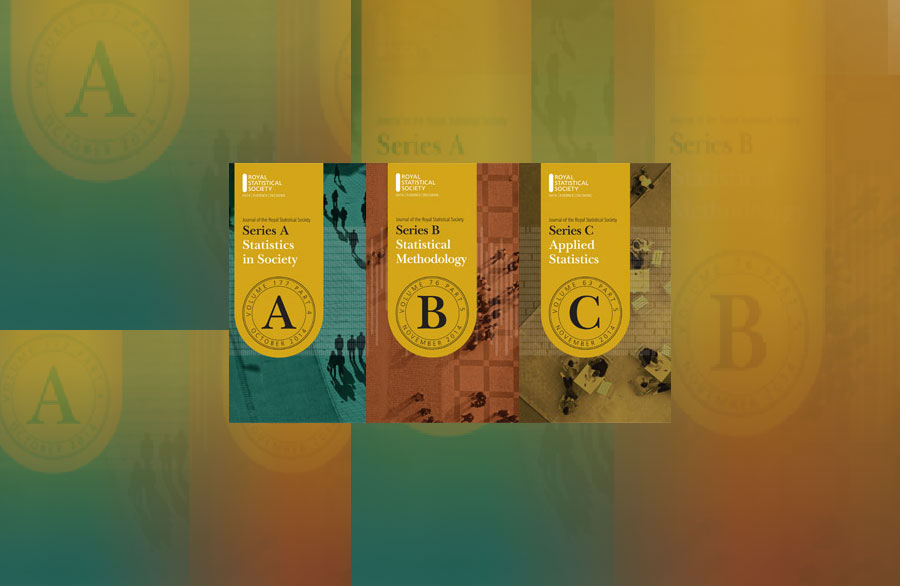# RSS journals all move up in impact factor rankings

All three journals of the RSS improved their impact factor scores and rankings in last week's announcement for 2019.

Series A (Statistics in society​) is now 2.210 (up from 1.785). The journal is now ranked 25/124 (statistics & probability category) and 12/51 (social sciences, mathematical methods).

Series B (Statistical methodology) is now 3.965 (was 3.278). The journal is now ranked 5/124 (statistics & probability).

Series C (Applied statistics) is now 1.590 (was 1.344). The journal is now ranked 43/124 (statistics & probability).